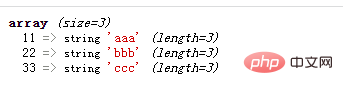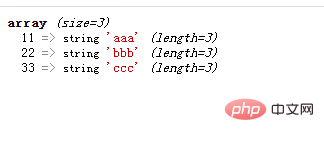# php数组中键和值间怎么转换位置php将数组中键和值转换位置

```<?php
\$arr1=array("aaa"=>11,"bbb"=>22,"ccc"=>33);
\$arr2=array();
foreach(\$arr1 as \$k=>\$v){
\$arr2[\$v]=\$k;
}
var_dump(\$arr2);
?>```array_flip()函数可以交换数组中的键和值

```<?php
\$arr1=array("aaa"=>11,"bbb"=>22,"ccc"=>33);
\$arr2=array_flip(\$arr1);
var_dump(\$arr2);
?>```1/1